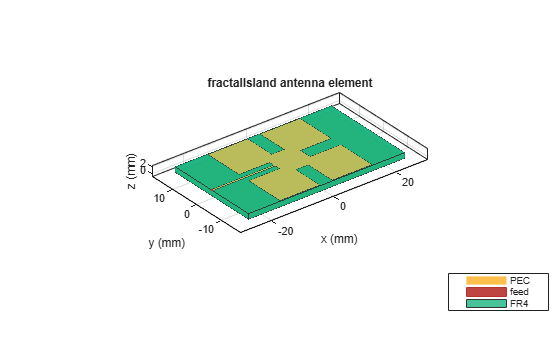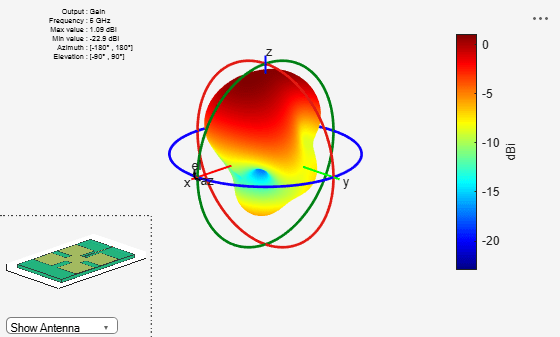# fractalIsland

Minkowski's loop fractal antenna

## Description

The `fractalIsland` object creates a Minkowski's loop fractal antenna. These fractal antennas are used in mobile phone and Wi-Fi® communications.A fractal antenna uses a self-similar design to maximize the length or increase the perimeter of a material that transmits or receives electromagnetic radiation within a given volume or area. The main advantage of fractal antennas is that they are compact, which is an important requirement for small and complex circuits. Fractal antennas also have more input impedance or resistance due to increased length or perimeter.

All fractal antennas are printed structures that are etched on a dielectric substrate.

## Creation

### Syntax

``ant = fractalIsland``
``ant = fractalIsland(Name,Value)``

### Description

example

````ant = fractalIsland` creates a Minkowski's loop fractal antenna. The default fractal is centered at the origin, and the number of iterations is set to 2. The length of the fractal is for an operating frequency of 6 GHz.```

example

````ant = fractalIsland(Name,Value)` sets properties using one or more name-value pairs. For example, `ant = fractalIsland('NumIterations',4)` creates a Minkowski's loop with four iterations.```

## Properties

expand all

Number of iterations performed on the fractal antenna, specified as a scalar integer.

Example: `'NumIterations',4`

Example: `ant.NumIterations = 4`

Data Types: `double`

Length of the fractal island along the x-axis, specified as a positive scalar integer in meters.

Example: `'Length',0.5000`

Example: `ant.Length = 0.5000`

Data Types: `double`

Width of the fractal island along the y-axis, specified as a positive scalar integer in meters.

Example: `'Width',0.0050`

Example: `ant.Width = 0.0050`

Data Types: `double`

Width of the feeding strip line, specified as a positive scalar integer in meters.

Example: `'StripLineWidth',3.0000e-04`

Example: `ant.StripLineWidth = 3.0000e-04`

Data Types: `double`

Length of the slot along the x-axis, specified as a positive scalar integer in meters.

Example: `'SlotLength',0.0050`

Example: `ant.SlotLength = 0.0050`

Data Types: `double`

Width of the slot along the y-axis, specified as a positive scalar integer in meters.

Example: `'SlotWidth',0.0050`

Example: `ant.SlotWidth = 0.0050`

Data Types: `double`

Height of the fractal above the ground plane along the z-axis, specified as a positive scalar integer in meters.

Example: `'Height',0.0034`

Example: `ant.Height = 0.0034`

Data Types: `double`

Type of dielectric material used as a substrate, specified as a dielectric object. For more information, see `dielectric`.

Example: `d = dielectric('FR4');` `ant = fractalIsland('Substrate',d)`

Example: `d = dielectric('FR4');` `ant = fractalIsland;` `ant.Substrate = d;`

Data Types: `string` | `char`

Length of the ground plane, specified as a positive scalar integer in meters.

Example: `'GroundPlaneLength',0.0550`

Example: `ant.GroundPlaneLength = 0.0550`

Data Types: `double`

Width of the ground plane, specified as a positive scalar integer in meters.

Example: `'GroundPlaneWidth',0.0550`

Example: `ant.GroundPlaneWidth = 0.0550`

Data Types: `double`

Signed distance of the fractal center from the origin, specified as a two-element real-valued vector with each element unit in meters.

Example: `'FractalCenterOffset',[0 0.080]`

Example: ```ant.FractalCenterOffset = [0 0.080]```

Data Types: `double`

Type of the metal used as a conductor, specified as a metal material object. You can choose any metal from the `MetalCatalog` or specify a metal of your choice. For more information, see `metal`. For more information on metal conductor meshing, see Meshing.

Example: ```m = metal('Copper'); 'Conductor',m```

Example: ```m = metal('Copper'); ant.Conductor = m```

Lumped elements added to the antenna feed, specified as a lumped element object. You can add a load anywhere on the surface of the antenna. By default, the load is at the origin. For more information, see `lumpedElement`.

Example: `'Load',lumpedelement`. `lumpedelement` is the object for the load created using `lumpedElement`.

Example: ```ant.Load = lumpedElement('Impedance',75)```

Tilt angle of the antenna, specified as a scalar or vector with each element unit in degrees. For more information, see Rotate Antennas and Arrays.

Example: `Tilt=90`

Example: `Tilt=[90 90]`,`TiltAxis=[0 1 0;0 1 1]` tilts the antenna at 90 degrees about the two axes defined by the vectors.

Note

The `wireStack` antenna object only accepts the dot method to change its properties.

Data Types: `double`

Tilt axis of the antenna, specified as:

• Three-element vector of Cartesian coordinates in meters. In this case, each coordinate in the vector starts at the origin and lies along the specified points on the X-, Y-, and Z-axes.

• Two points in space, each specified as three-element vectors of Cartesian coordinates. In this case, the antenna rotates around the line joining the two points in space.

• A string input describing simple rotations around one of the principal axes, 'X', 'Y', or 'Z'.

For more information, see Rotate Antennas and Arrays.

Example: `TiltAxis=[0 1 0]`

Example: `TiltAxis=[0 0 0;0 1 0]`

Example: `TiltAxis = 'Z'`

Note

The `wireStack` antenna object only accepts the dot method to change its properties.

Data Types: `double`

## Object Functions

 `show` Display antenna, array structures or shapes `axialRatio` Axial ratio of antenna `beamwidth` Beamwidth of antenna `charge` Charge distribution on antenna or array surface `current` Current distribution on antenna or array surface `design` Design prototype antenna or arrays for resonance around specified frequency `efficiency` Radiation efficiency of antenna `EHfields` Electric and magnetic fields of antennas; Embedded electric and magnetic fields of antenna element in arrays `impedance` Input impedance of antenna; scan impedance of array `mesh` Mesh properties of metal, dielectric antenna, or array structure `meshconfig` Change mesh mode of antenna structure `optimize` Optimize antenna or array using SADEA optimizer `pattern` Radiation pattern and phase of antenna or array; Embedded pattern of antenna element in array `patternAzimuth` Azimuth pattern of antenna or array `patternElevation` Elevation pattern of antenna or array `rcs` Calculate and plot radar cross section (RCS) of platform, antenna, or array `returnLoss` Return loss of antenna; scan return loss of array `sparameters` Calculate S-parameter for antenna and antenna array objects `vswr` Voltage standing wave ratio of antenna

## Examples

collapse all

Create and view a Minkowski's loop fractal antenna with default property values.

`ant = fractalIsland`
```ant = fractalIsland with properties: NumIterations: 2 Length: 0.0295 Width: 0.0295 StripLineWidth: 6.0000e-04 SlotLength: 0.0040 SlotWidth: 0.0040 Height: 0.0016 Substrate: [1x1 dielectric] GroundPlaneLength: 0.0500 GroundPlaneWidth: 0.0300 FractalCenterOffset: [0 0] Conductor: [1x1 metal] Tilt: 0 TiltAxis: [1 0 0] Load: [1x1 lumpedElement] ```
`show(ant)`Create and view a Minkowski's loop fractal antenna on FR4 substrate.

```ant = fractalIsland('Substrate',dielectric('FR4')); show(ant)```Plot the radiation pattern of the antenna at a frequency of 6 GHz.

`pattern(ant,6e9)`## Version History

Introduced in R2019a